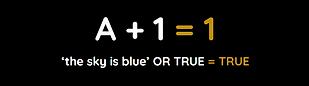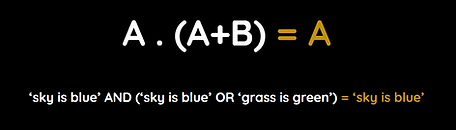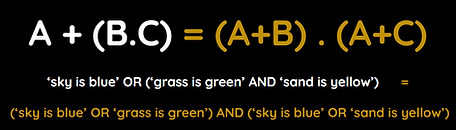top of page

## 2020 +

Boolean algebra is used to simplify Boolean expressions so that they are easier to understand.

Because calculations can use dozens of logical operators, they are simplified in Boolean Algebra using symbols rather than words.

Take your time and don't panic. In an exam, you might get a list of identities (rules) to use.

One tip to solving boolean algebra is to imagine that A and B are real expressions.

A represents the true statement 'the sky is blue'

B represents the true statement 'grass is green'

0 always means FALSE

1 always means TRUE

Boolean Symbols

A = NOT A

A . B = A AND B

A + B = A OR B

Boolean Identities are the rules that are used to simplify Boolean expressions.

Each identity (law) has an AND form and an OR form, depending on whether AND or OR is being used.

## Commutative LawAND form:OR form:

This law just switches the order of the expressions

For example, 'sky is blue' AND 'grass is green' makes logical sense in either order.

## Idempotent Law

=AND form:OR form:

This law removes repetition

## Complement Law

NOTAND form:

The sky cannot be blue and not blue at the same time, so it must be 0 (FALSE).OR form:

The sky is blue or not blue must be 1 (TRUE) as it has to be one of these options.

## Identity LawAND form:

1 represents TRUE. Both statements are true so it can be simplified as just A.OR form:

0 represents FALSE. Because A is true, you can ignore the false statement and it can be simplified as just A.

## Annulment LawAND form:

0 represents FALSE. Even though A is true, a statement cannot be true and false at the same time, so it must be 0 (FALSE).OR form:

1 represents TRUE. Both statements are true so this can be simplified as just 1 (TRUE).

## Absorption LawAND form:OR form:

Absorption law reduces a bracket into one value.

If the first A is true then both values in the brackets are true but if the first A is false then both values are false. Therefore this equation relies entirely on A and can be simplified as just A.

## Association Law

(   )AND form:OR form:

This law separates a bracketed expression that uses the same operator inside and outside the brackets by removing the brackets.

## Distribution Law

( ) = ( ) ( )

AND form:OR form:The value outside of the bracket (e.g. A) is multiplied by both values inside the brackets, forming two new brackets which are linked by the logical operator formerly within the bracket.

Notice that the logical operator role is switched, e.g. AND switches from within the brackets, to between the new brackets.

A note about distribution law - The three values may not necessarily be three separate letters (e.g. A, B and C) as B or C could be NOT A for example. A NOT value is considered a new value, e.g. A and Ā are separate values.

Another note about distribution law - Exam questions may ask you to perform the distribution law (or any law) in reverse.
For example, converting (A+B) . (A+C) into A + (B.C)

## Boolean Algebra Exam Question

Some previous exam questions have listed helpful laws for you but others haven't, so you should know each individual law.

In a previous exam, the candidates were given three general laws to help them. P, Q and R just represent three different values.​

P . 1 = P (Identity Law)

P . Q  + P . R = P. (Q + R) (Distribution Law)

P + P = 1 (Complement Law)

Using the rules above, candidates were asked to simplify the following expression:

X = A . B + A . B

The general laws have been given to you for a reason.

You need to look at the laws provided and see which one currently matches the expression in front of you.

If you look closely in this example, the second law is very similar to the expression you are asked to simplify so you can use it to make the first simplification, just swap P for A, Q for B and R for NOT B:

Using this law

P . Q  + P . R = P. (Q + R)​

X = A . B + A . B

simplifies as:

X = A . (B + B)

Now you need to see which of the three provided laws can be used with the current expression.

The third law is very similar to the expression you now need to simplify further, just swap P for B and NOT P for NOT B:

Using this law

P + P = 1

X = A . (B + B)

simplifies as:

X = A . (1)

And finally, there is one law left to use.

The first law is very similar to the expression you now need to simplify further, just swap P for A.

Using this law

P . 1 = P

X = A . (1)

simplifies as:

X = A

You have now used all three laws and the expression is fully simplified.

Remember - Look at the laws that you have been given and see which law matches your expression## Questo's Questions

2.2 - Boolean Algebra:

1. Draw the example equations and write a brief description of each of the eight Boolean laws:

• Commutative Law

• Idempotent Law

• Complement Law

• Identity Law

• Annulment Law

• Absorption Law

• Associate Law

• Distributive Law

2. Below are three Boolean identities:

P . P = 0

(P + Q) . R = (P . R) + (Q . R)

P + 0 = P

Using the three rules above, simplify the following expression:

X = (A + B) . Ā​

This law is called 'Inverse Law' in the Eduqas 2016 teacher guidance but 'Complement Law' in the 2020 specification.

This law is called 'Zero and One Law' in the Eduqas 2016 teacher guidance but 'Annulment Law' in the 2020 specification.

This law is called 'Associate Law' in the Eduqas 2016 teacher guidance but 'Association Law' in the 2020 specification.

This law is called 'Distributive Law' in the Eduqas 2016 teacher guidance but 'Distribution Law' in the 2020 specification.

bottom of page